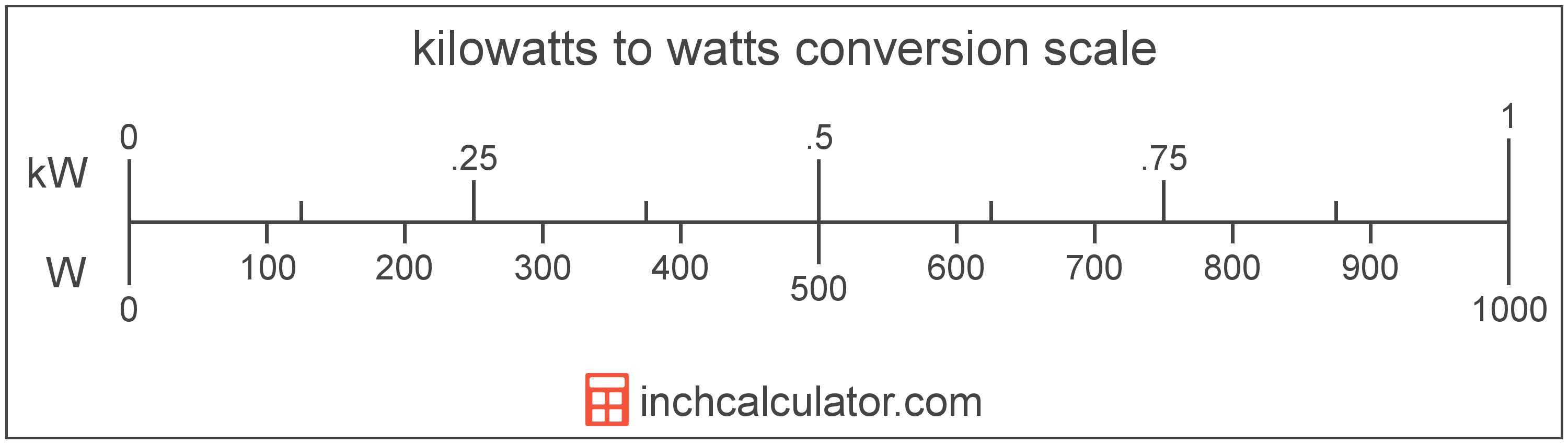# Watts to Kilowatts Conversion

Enter the power in watts below to get the value converted to kilowatts.

Results in Kilowatts:1,000 W = 1 kW
Do you want to convert kilowatts to watts?

## How to Convert Watts to KilowattsTo convert a watt measurement to a kilowatt measurement, divide the power by the conversion ratio.

Since one kilowatt is equal to 1,000 watts, you can use this simple formula to convert:

kilowatts = watts ÷ 1,000

The power in kilowatts is equal to the watts divided by 1,000.

For example, here's how to convert 5,000 watts to kilowatts using the formula above.
5,000 W = (5,000 ÷ 1,000) = 5 kW

## Watts

One watt is the power equal to the rate of energy of one joule per second in an electrical circuit. One watt is also equal to the power produced in a circuit with a voltage potential of one volt with a current of one amp.

The watt is the SI derived unit for power in the metric system. Watts can be abbreviated as W; for example, 1 watt can be written as 1 W.

Watt's Law states the relationship between power, current, and voltage. Using Watt's Law, it's possible to express the power in watts as an expression using current and voltage.

PW = IA × VV

The power in watts is equal to the current in amperes times the potential difference in volts.

Combining this with Ohm's Law, it's also possible to express the power in watts using resistance in addition to volts and amps.

## Kilowatts

One kilowatt is the power equal to 1,000 watts, or the energy consumption at a rate of 1,000 joules per second.

The kilowatt is a multiple of the watt, which is the SI derived unit for power. In the metric system, "kilo" is the prefix for 103. Kilowatts can be abbreviated as kW; for example, 1 kilowatt can be written as 1 kW.

## Watt to Kilowatt Conversion Table

Watt measurements converted to kilowatts
Watts Kilowatts
1 W 0.001 kW
2 W 0.002 kW
3 W 0.003 kW
4 W 0.004 kW
5 W 0.005 kW
6 W 0.006 kW
7 W 0.007 kW
8 W 0.008 kW
9 W 0.009 kW
10 W 0.01 kW
20 W 0.02 kW
30 W 0.03 kW
40 W 0.04 kW
50 W 0.05 kW
60 W 0.06 kW
70 W 0.07 kW
80 W 0.08 kW
90 W 0.09 kW
100 W 0.1 kW
200 W 0.2 kW
300 W 0.3 kW
400 W 0.4 kW
500 W 0.5 kW
600 W 0.6 kW
700 W 0.7 kW
800 W 0.8 kW
900 W 0.9 kW
1,000 W 1 kW

## References

1. International Bureau of Weights and Measures, The International System of Units, 9th Edition, 2019, https://www.bipm.org/documents/20126/41483022/SI-Brochure-9-EN.pdf